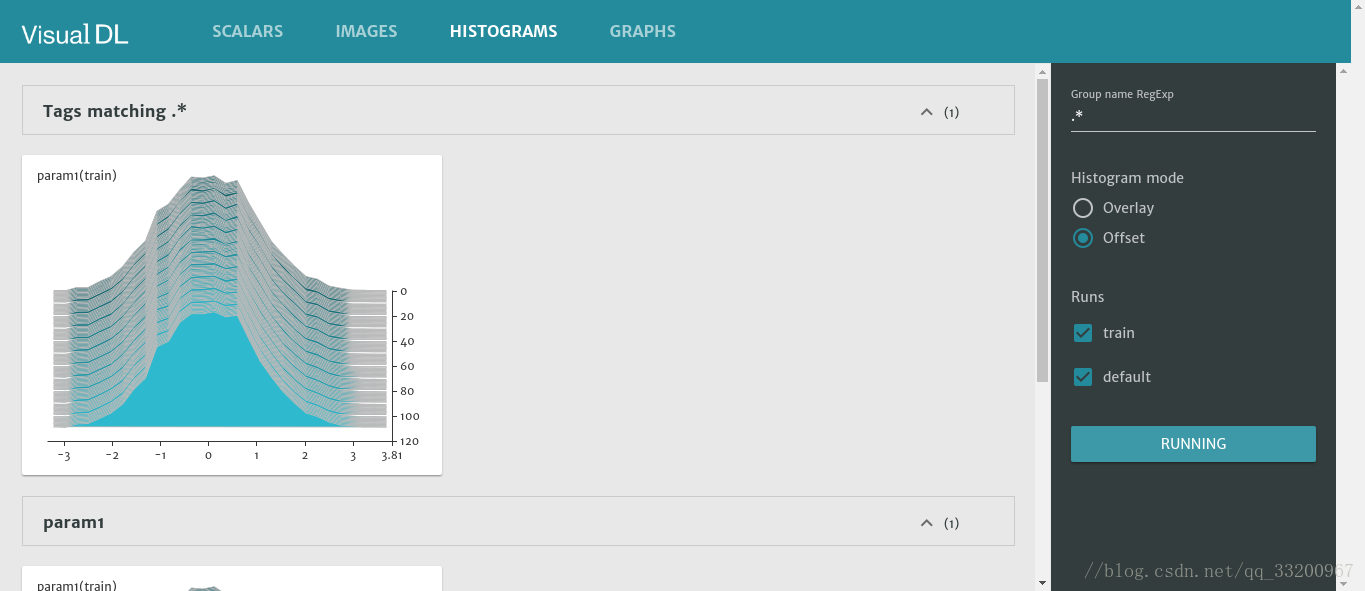# 前言

VisualDL 是一个面向深度学习任务设计的可视化工具，包含了 scalar、参数分布、模型结构、图像可视化等功能。可以这样说：“所见即所得”。我们可以借助 VisualDL 来观察我们训练的情况，方便我们对训练的模型进行分析，改善模型的收敛情况。

1. `scalar`，趋势图，可用于训练测试误差的展示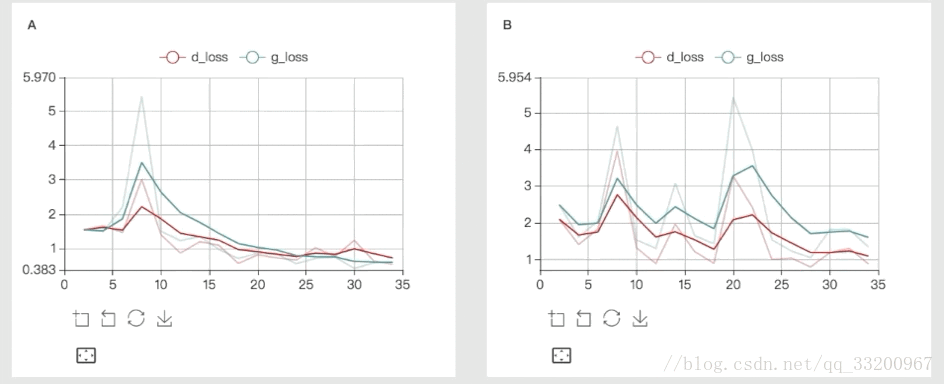2. `image`, 图片的可视化，可用于卷积层或者其他参数的图形化展示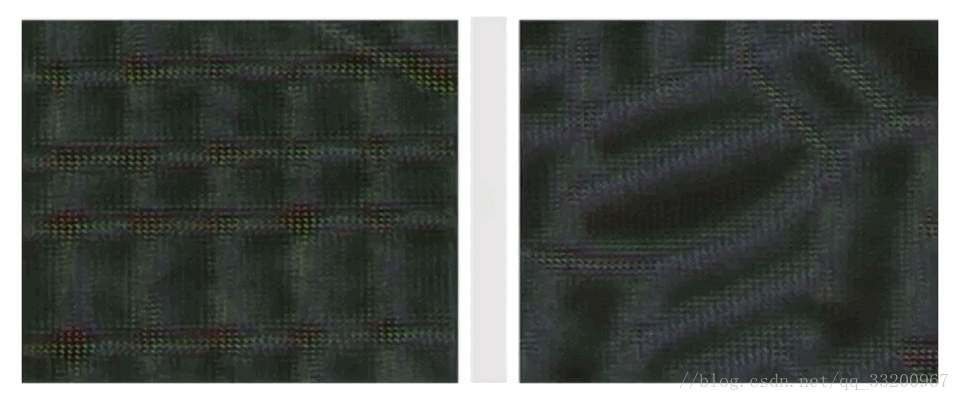3. `histogram`, 用于参数分布及变化趋势的展示4. `graph`，用于训练模型结构的可视化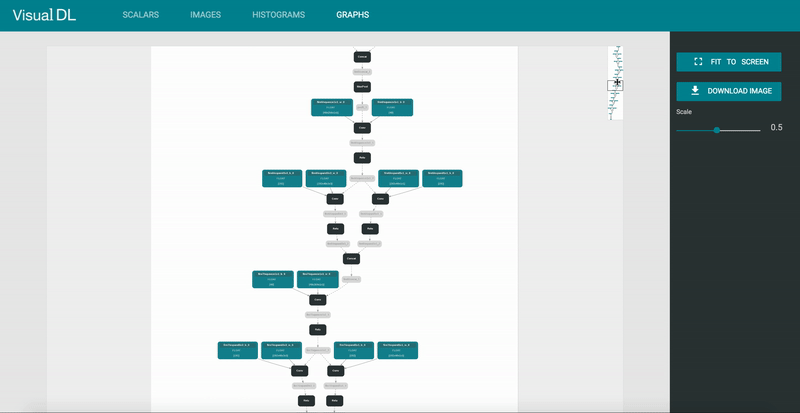以上的图像来自 VisualDL 的 Github

# VisualDL 的安装

## 使用 pip 安装

``````pip install --upgrade visualdl
``````

``````# 在当前位置下载一个日志
vdl_create_scratch_log
# 如果提示命令不存在，那就使用下面这条命令
vdl_scratch.py
``````

``````visualdl --logdir ./scratch_log --port 8080
``````

• `host` 设定 IP
• `port` 设定端口
• `model_pb` 指定 ONNX 格式的模型文件，这木方我们还没要用到

``````root@test:/home/test/VisualDL# visualdl --logdir ./scratch_log --port 8080
Traceback (most recent call last):
File "/usr/local/bin/visualdl", line 29, in <module>
import visualdl.server.graph as vdl_graph
File "/usr/local/lib/python2.7/dist-packages/visualdl/server/graph.py", line 23, in <module>
from . import onnx
File "/usr/local/lib/python2.7/dist-packages/visualdl/server/onnx/__init__.py", line 8, in <module>
from .onnx_pb2 import ModelProto
File "/usr/local/lib/python2.7/dist-packages/visualdl/server/onnx/onnx_pb2.py", line 213, in <module>
options=None, file=DESCRIPTOR),
TypeError: __init__() got an unexpected keyword argument 'file'
``````

Protobuf 的版本要不小于 3.5.0，如何小于这个版本可以使用以下命令升级：

``````pip install protobuf -U
``````

``````http://127.0.0.1:8080
``````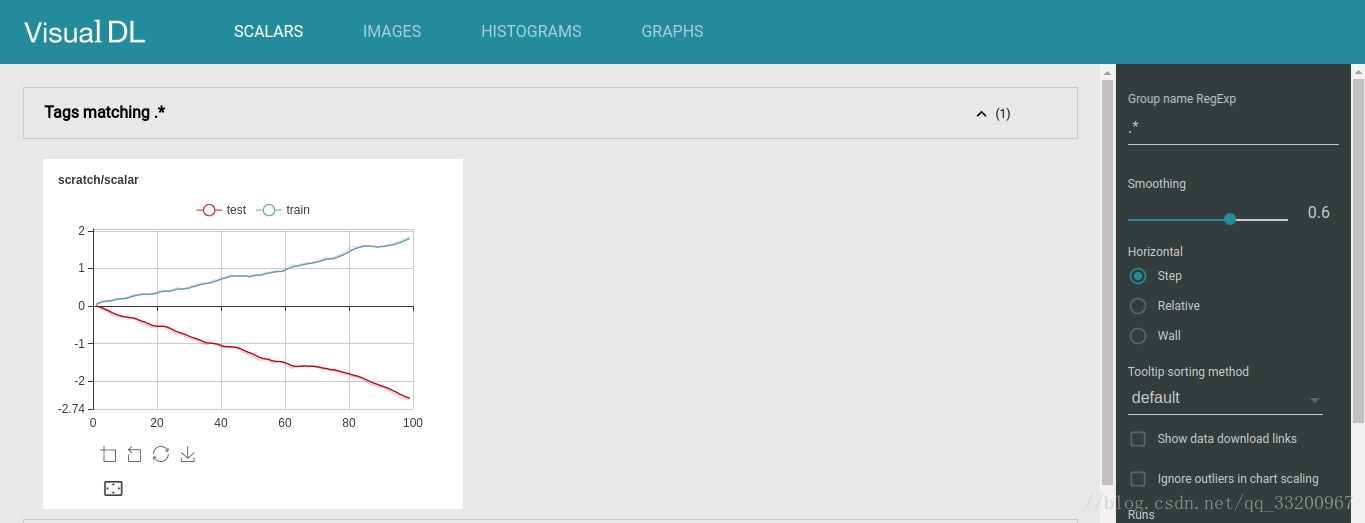## 使用源码安装

``````# 安装npm
apt install npm
# 安装node
apt install nodejs-legacy
# 安装cmake
apt install cmake
# 安装unzip
apt install unzip
``````

``````git clone https://github.com/PaddlePaddle/VisualDL.git
cd VisualDL
``````

``````python setup.py bdist_wheel
``````

``````pip install --upgrade dist/visualdl-*.whl
``````

# 简单使用 VisualDL

``````# coding=utf-8
# 导入VisualDL的包
from visualdl import LogWriter

# 创建一个LogWriter，第一个参数是指定存放数据的路径，
# 第二个参数是指定多少次写操作执行一次内存到磁盘的数据持久化
logw = LogWriter("./random_log", sync_cycle=10000)

# 创建训练和测试的scalar图，
# mode是标注线条的名称，
# scalar标注的是指定这个组件的tag
with logw.mode('train') as logger:
scalar0 = logger.scalar("scratch/scalar")

with logw.mode('test') as logger:
scalar1 = logger.scalar("scratch/scalar")

# 读取数据
for step in range(1000):
scalar0.add_record(step, step * 1. / 1000)
scalar1.add_record(step, 1. - step * 1. / 1000)
``````

``````visualDL --logdir ./random_log --port 8080
``````

``````http://127.0.0.1:8080
``````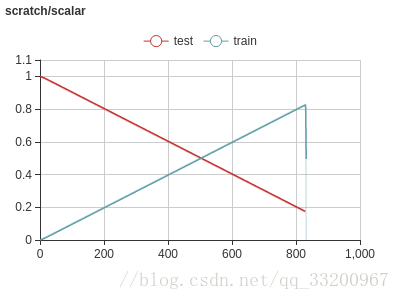## 定义 VisualDL 组件

``````# 创建VisualDL，并指定当前该项目的VisualDL的路径
logdir = "../data/tmp"
logwriter = LogWriter(logdir, sync_cycle=10)

# 创建loss的趋势图
with logwriter.mode("train") as writer:
loss_scalar = writer.scalar("loss")

# 创建acc的趋势图
with logwriter.mode("train") as writer:
acc_scalar = writer.scalar("acc")

# 定义没多少次重新输出一遍
num_samples = 4
# 创建卷积层和输出图像的图形化展示
with logwriter.mode("train") as writer:
conv_image = writer.image("conv_image", num_samples, 1)
input_image = writer.image("input_image", num_samples, 1)

# 创建可视化的训练模型结构
with logwriter.mode("train") as writer:
param1_histgram = writer.histogram("param1", 100)
``````

``````# 定义图像的类别数量
class_dim = 10
# 定义图像的通道数和大小
image_shape = [3, 32, 32]
# 定义输入数据大小，指定图像的形状，数据类型是浮点型
image = fluid.layers.data(name='image', shape=image_shape, dtype='float32')
# 定义标签，类型是整型
label = fluid.layers.data(name='label', shape=, dtype='int64')
``````

``````# 获取神经网络
net, conv1 = vgg16_bn_drop(image)
# 获取全连接输出，获得分类器
predict = fluid.layers.fc(
input=net,
size=class_dim,
act='softmax',
param_attr=ParamAttr(name="param1", initializer=NormalInitializer()))
``````

``````# 获取损失函数
cost = fluid.layers.cross_entropy(input=predict, label=label)
# 定义平均损失函数
avg_cost = fluid.layers.mean(x=cost)

# 每个batch计算的时候能取到当前batch里面样本的个数，从而来求平均的准确率
batch_size = fluid.layers.create_tensor(dtype='int64')
print batch_size
batch_acc = fluid.layers.accuracy(input=predict, label=label, total=batch_size)

# 定义优化方法
optimizer = fluid.optimizer.Momentum(
learning_rate=learning_rate,
momentum=0.9,
regularization=fluid.regularizer.L2Decay(5 * 1e-5))

opts = optimizer.minimize(avg_cost)
``````

``````# 是否使用GPU
place = fluid.CUDAPlace(0) if use_cuda else fluid.CPUPlace()
# 创建调试器
exe = fluid.Executor(place)
# 初始化调试器
exe.run(fluid.default_startup_program())
``````

``````# 获取训练数据

# 指定数据和label的对于关系
feeder = fluid.DataFeeder(place=place, feed_list=[image, label])
``````

``````step = 0
sample_num = 0
start_up_program = framework.default_startup_program()
param1_var = start_up_program.global_block().var("param1")
``````

``````accuracy = fluid.average.WeightedAverage()
# 开始训练，使用循环的方式来指定训多少个Pass
for pass_id in range(num_passes):
# 从训练数据中按照一个个batch来读取数据
accuracy.reset()
loss, conv1_out, param1, acc, weight = exe.run(fluid.default_main_program(),
feed=feeder.feed(data),
fetch_list=[avg_cost, conv1, param1_var, batch_acc,
batch_size])
pass_acc = accuracy.eval()
``````

## 把数据都添加到 VisualDL

``````# 重新启动图形化展示组件
if sample_num == 0:
input_image.start_sampling()
conv_image.start_sampling()
# 获取taken
idx1 = input_image.is_sample_taken()
idx2 = conv_image.is_sample_taken()
# 保证它们的taken是一样的
assert idx1 == idx2
idx = idx1
if idx != -1:
# 加载输入图像的数据数据
image_data = data
input_image_data = np.transpose(
image_data.reshape(image_shape), axes=[1, 2, 0])
input_image.set_sample(idx, input_image_data.shape,
input_image_data.flatten())
# 加载卷积数据
conv_image_data = conv1_out
conv_image.set_sample(idx, conv_image_data.shape,
conv_image_data.flatten())

# 完成输出一次
sample_num += 1
if sample_num % num_samples == 0:
input_image.finish_sampling()
conv_image.finish_sampling()
sample_num = 0
``````

``````# 加载趋势图的数据
``````

``````# 添加模型结构数据
``````

``````loss:[16.7996] acc:[0.0703125] pass_acc:[0.0703125]
loss:[15.192436] acc:[0.1171875] pass_acc:[0.09375]
loss:[14.519127] acc:[0.109375] pass_acc:[0.09895833]
loss:[15.262356] acc:[0.125] pass_acc:[0.10546875]
loss:[13.626783] acc:[0.078125] pass_acc:[0.1]
loss:[11.8960285] acc:[0.09375] pass_acc:[0.09895833]
``````

``````visualDL --logdir ./tmp --port 8080
``````

``````http://127.0.0.1:8080
``````

1. 我们训练的趋势图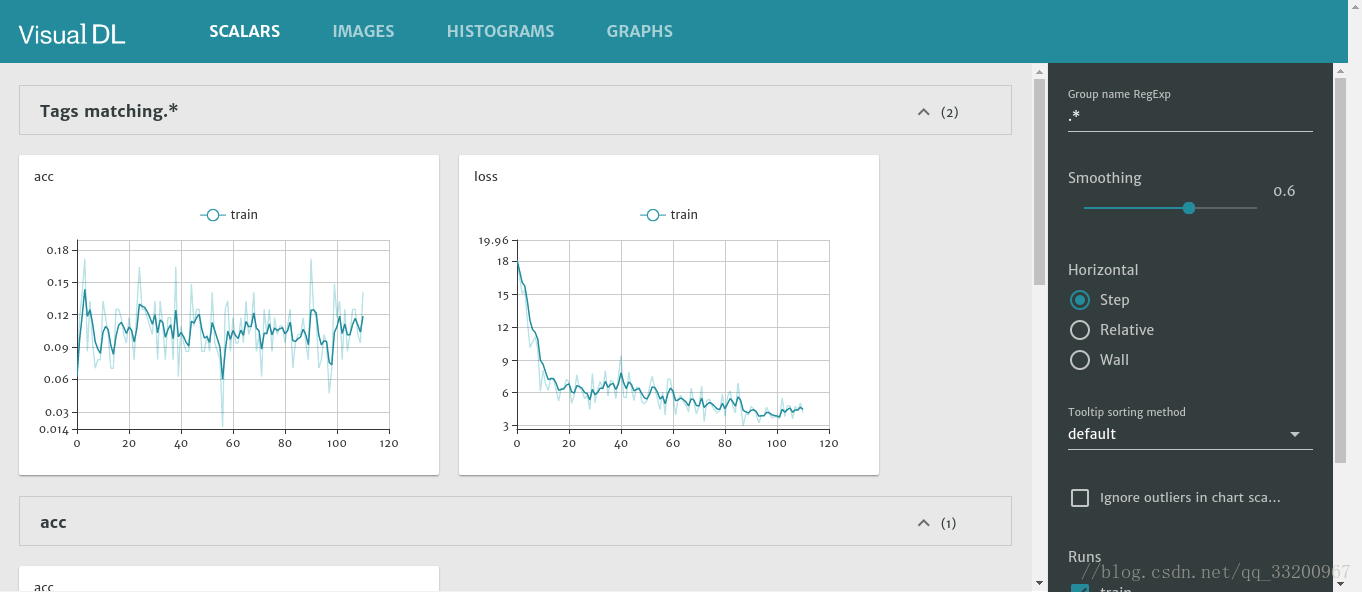2. 卷积和输入图像的可视化页面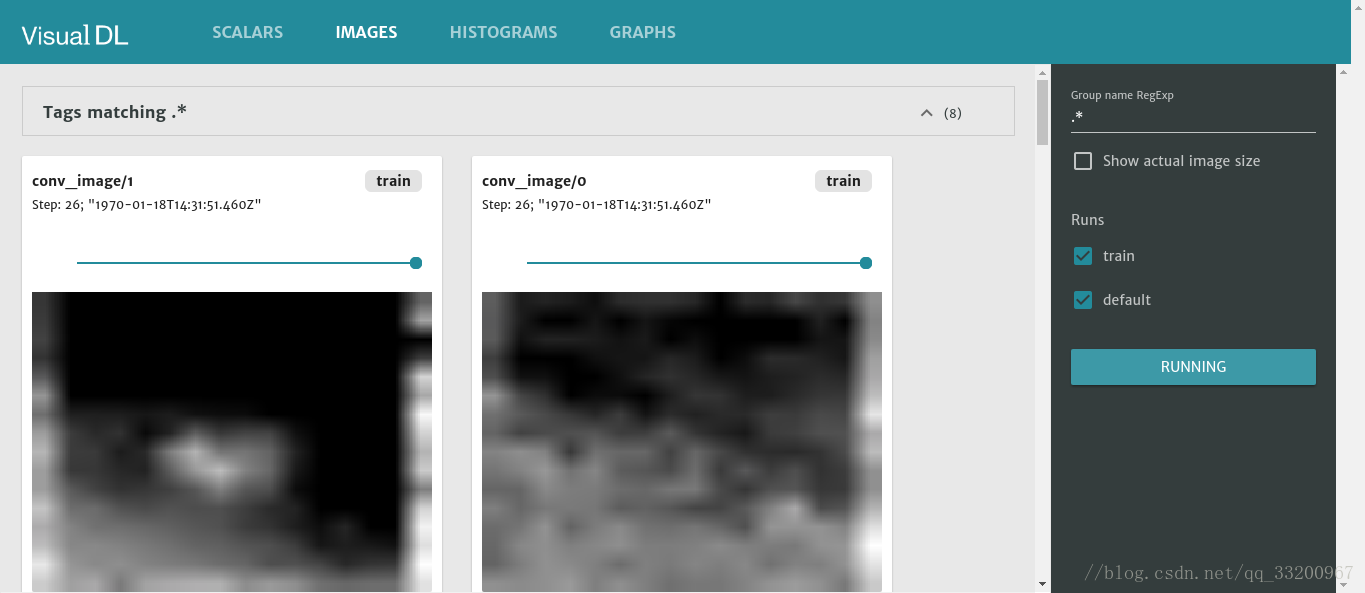3. 训练参数的变化情况